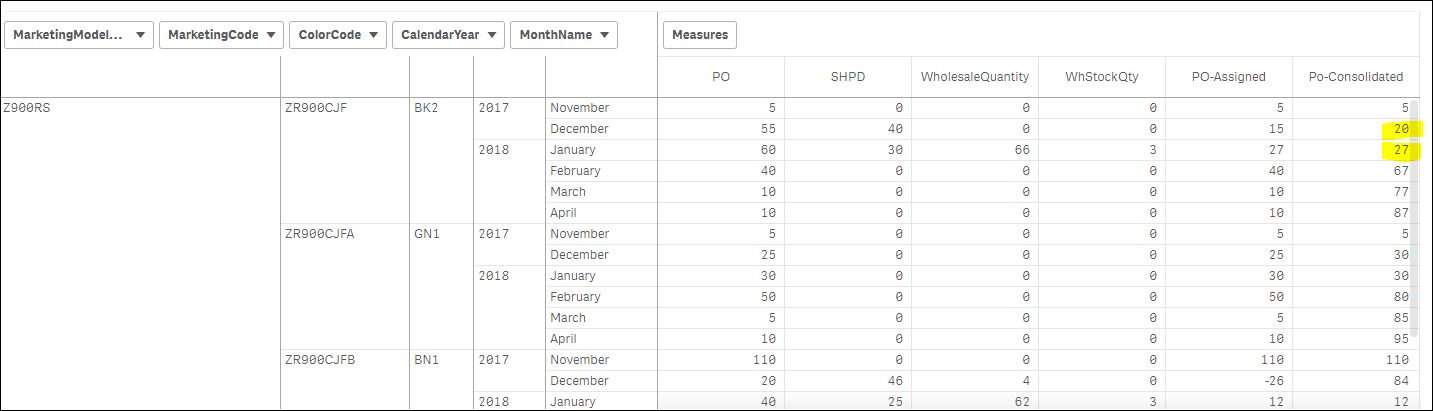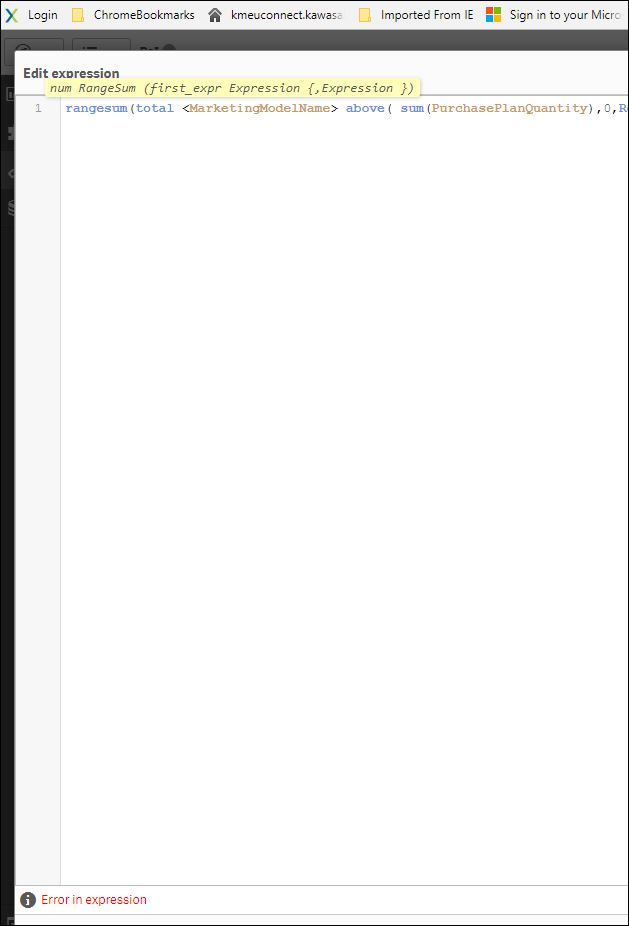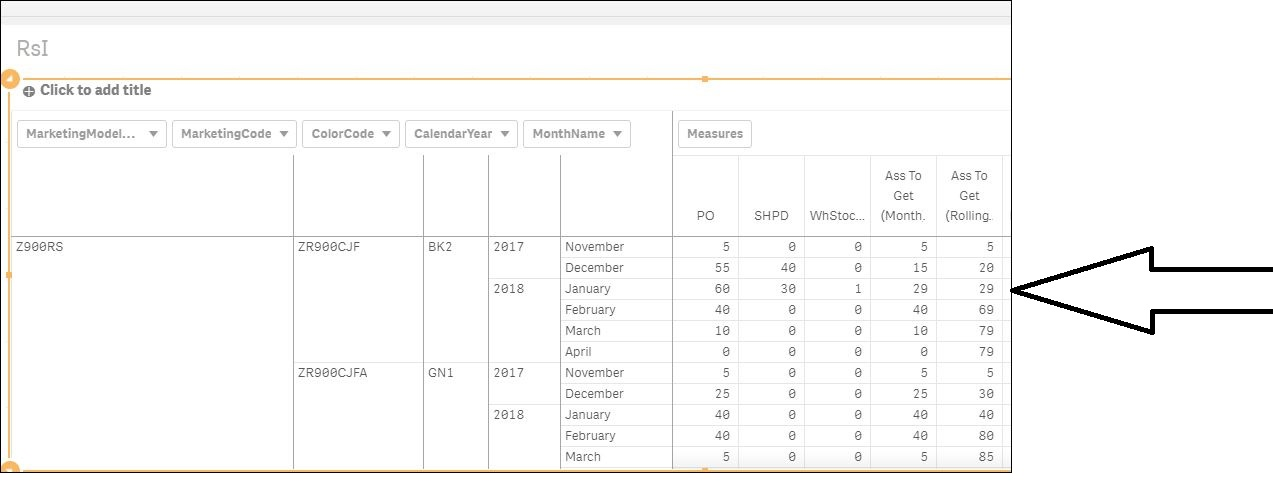# App Development

Announcements
Qlik Insider: SaaS capabilities for Data Integration and Analytics, May 25th! Register Now
cancel
Showing results for
Did you mean:Contributor III

## Rangesum question

Hi Guys,

I've got a rangesum function in a pivot table which I can't make it work as I need.

This is the formula I'm using, it works fine till i keep CalendarYear dimension out of the pivot table, once I put it inside the pivot, the  rolling is interrupted at any new calendar year:The January yellow highlighted value should be 47 instead , just as in the following screen shot, with no calendar year1 Solution

Accepted SolutionsEmployee

I assume that the column TestMsr is the one that we're looking at. But since you changed the name of the column, you need to change this in the expression to reflect that.

The expression

Rangesum(Above( total [Po-Consolidated] ) , X )

will take the value of Po-Consolidated from the line above, and add X. But now you instead want the value of TestMsr from the line above.

So, then you need to change the expression to

If(

ColorCode=Above(total ColorCode),

Rangesum(Above( total [TestMsr] ) , Sum(PurchasePlanQuantity), -Sum(ShippedQty), -Sum(WhStockQty) ),

RangeSum( Sum(PurchasePlanQuantity), -Sum(ShippedQty), -Sum(WhStockQty))

)

HIC

15 RepliesEmployee

Use the total qualifier:

RangeSum( total <expression> )

Above( total <expression> )

HICMVP

Hi Henric -

I have never seen this syntax before. I have seen TOTAL within Above() function, but never seen RangeSum() with TOTAL. Also, TOTAL within Above() doesn't really allow to use field names between <>. But it seems that RangeSum() does. Do you have a blog where we can read more about this?

Thanks,

SunnyContributor III
Author

I forgot to paste the formula I'm using:

rangesum( above( sum(PurchasePlanQuantity),0,RowNo()+12) )-(rangesum( above( sum(ShippedQty),0,RowNo()+12) )+rangesum( above( sum(WhStockQty),0,RowNo()+12) ))Contributor III
Author

Hi Henric, thanks for your answer, I tried and put a total in the editor, but qlik doesn't allow the total predicateMVP

This is what confuses me... I have never seen TOTAL within RangeSum, but if Henric has mentioned about this, I would think that we are probably missing something.Employee

Sunny

My bad. Temporary brain drop... The total qualifier should of course be in the Above() function.

Also, it won't work with field specifiers after the total qualifier. So the work around must be a conditional evaluation like

If(Year=Above(total Year),Above(total Sum(Sales)),0)

HICEmployee

Try

If(

Year=Above(total Year),

Rangesum(Above( total [Po-Consolidated] ) , Sum(PurchasePlanQuantity), -Sum(ShippedQty), -Sum(WhStockQty) ),

RangeSum( Sum(PurchasePlanQuantity), -Sum(ShippedQty), -Sum(WhStockQty))

)MVP

Never thought of using this work around, this is amazing. Thanks for sharing this.

Best,

SunnyContributor III
Author

Hi Enric, awesome script but it's still giving me what I've got already. The range sum of the Calendar Year dimension, I'm trying on the other hand to include the above calendar year dimension into the current calendar year dimension.

The 29 units to which the arrow is pointing should be 49 insteadTags